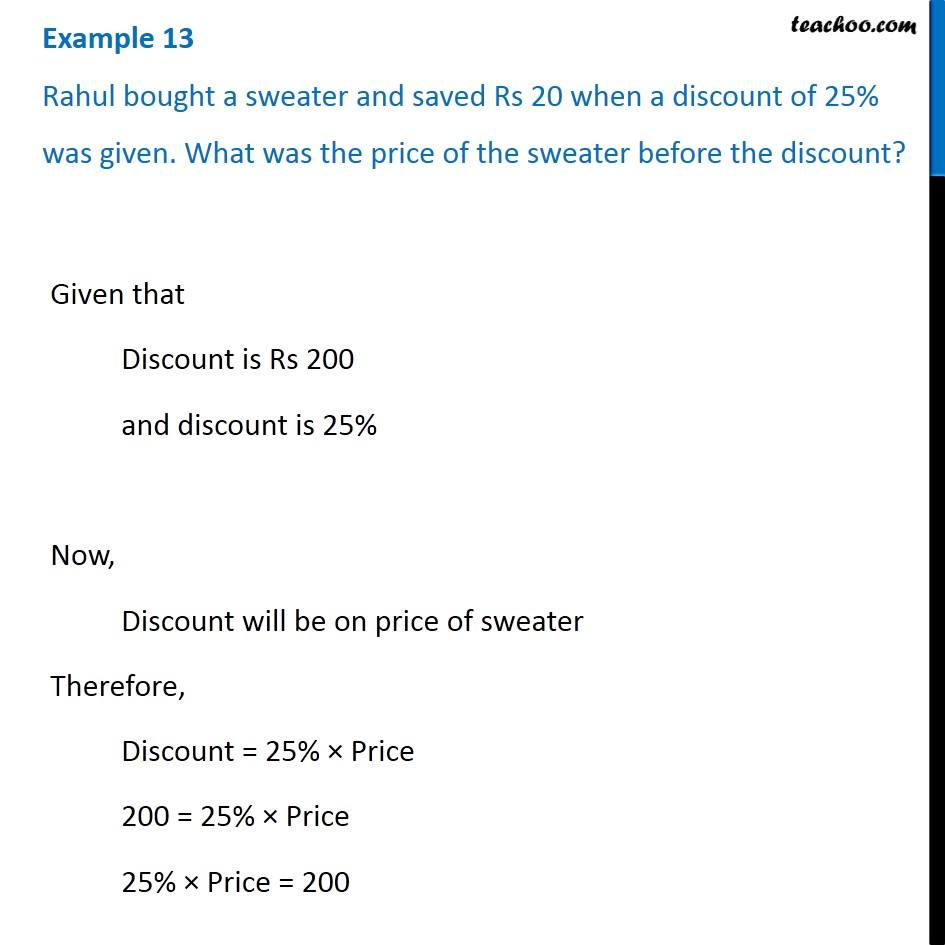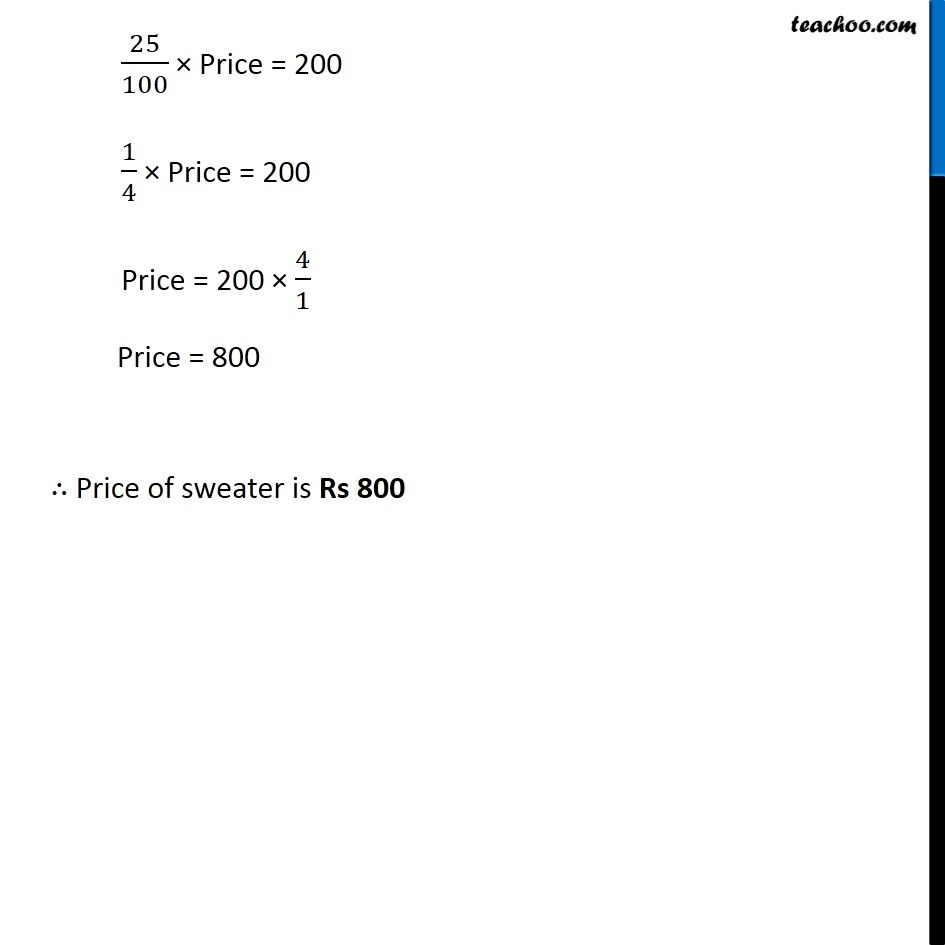Subscribe to our Youtube Channel - https://you.tube/teachoo

1. Chapter 8 Class 7 Comparing Quantities
2. Concept wise
3. Using percentage

Transcript

Example 13 Rahul bought a sweater and saved Rs 20 when a discount of 25% was given. What was the price of the sweater before the discount? Given that Discount is Rs 200 and discount is 25% Now, Discount will be on price of sweater Therefore, Discount = 25% × Price 200 = 25% × Price 25% × Price = 200 25/100 × Price = 200 1/4 × Price = 200 Price = 200 × 4/1 Price = 800 ∴ Price of sweater is Rs 800

Using percentage